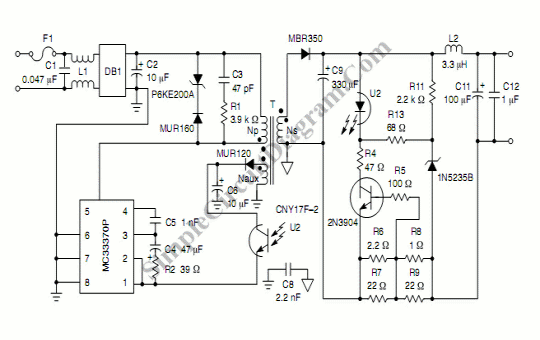# Constant Current Constant Voltage Switching Power Supply

This is a circuit that produces constant current constant voltage  SMPS. To efficiently charge a battery, we can use constant current constant voltage  SMPS. This circuit produces 600 mA at constant current mode and 7.2V at constant voltage mode. Here is the circuit:The operation of this circuit is simple, When the load impedance is low, then the output current will increase. The output voltage would be constant at 7.2V, when the load impedance is high. The output voltage unaffected by any load impedance, the voltage will be constant, as long as the load draws no more than 600 mA current (12 ohm load). The constant current mode will work, when the the load recieves more current than 600 mA (the impedance falls below 12 ohm). So the current will be constant. To keep the current constant at 600 mA  the output voltage will drop below 7.2 volt. It will happen if the load impedance get lower than 12 ohm.

Transformer:

Core Type: E13/7/4
Bobbin: B66202 from Siemens or equivalent
Ferrite Material: N67 from Siemens or equivalent
Secondary: 8 turns of #26 AWG, triple insulated wire
Gap: 0.137 mm for a primary inductance of 2.002 mH
Primary: 133 turns of #35 AWG, three layers 0.002” mylar tape
Auxiliary: 27 turns of #38 AWG, two layers 0.002” mylar tape

Inductor, L1:

22 mH (Siemens) Common–mode choke

[Schematic diagram source: ON Semiconductor Application Notes]